# Page Rank

#### Page RankMathematical PageRanks (out of 100) for a simple network (PageRanks reported by Google are rescaled logarithmically). Page C has a higher PageRank than Page E, even though it has fewer links to it; the link it has is of a much higher value. A web surfer who chooses a random link on every page (but with 15% likelihood jumps to a random page on the whole web) is going to be on Page E for 8.1% of the time. (The 15% likelihood of jumping to an arbitrary page corresponds to a damping factor of 85%.) Without damping, all web surfers would eventually end up on Pages A, B, or C, and all other pages would have PageRank zero. Page A is assumed to link to all pages in the web, because it has no outgoing links.

PageRank is a link analysis algorithm, named after Larry Page, used by the Google Internet search engine that assigns a numerical weighting to each element of a hyperlinked set of documents, such as the World Wide Web, with the purpose of “measuring” its relative importance within the set. The algorithm may be applied to any collection of entities with reciprocal quotations and references. The numerical weight that it assigns to any given element E is also called the PageRank of E and denoted by PR(E).

The name “PageRank” is a trademark of Google, and the PageRank process has been patented (U.S. Patent 6,285,999). However, the patent is assigned to Stanford University and not to Google. Google has exclusive license rights on the patent from Stanford University. The university received 1.8 million shares of Google in exchange for use of the patent; the shares were sold in 2005 for \$336 million.

### Description

 “ PageRank reflects our view of the importance of web pages by considering more than 500 million variables and 2 billion terms. Pages that we believe are important pages receive a higher PageRank and are more likely to appear at the top of the search results.PageRank also considers the importance of each page that casts a vote, as votes from some pages are considered to have greater value, thus giving the linked page greater value. We have always taken a pragmatic approach to help improve search quality and create useful products, and our technology uses the collective intelligence of the web to determine a page’s importance. ”

In other words, a PageRank results from a “ballot” among all the other pages on the World Wide Web about how important a page is. A hyperlink to a page counts as a vote of support. The PageRank of a page is defined recursively and depends on the number and PageRank metric of all pages that link to it (“incoming links“). A page that is linked to by many pages with high PageRank receives a high rank itself. If there are no links to a web page there is no support for that page.

Google assigns a numeric weighting from 0-10 (but 0 is used just for penalized or non analyzed-pages) for each webpage on the Internet; this PageRank denotes a site’s importance in the eyes of Google. The PageRank is derived from a theoretical probability value on a logarithmic scale like the Richter Scale. The PageRank of a particular page is roughly based upon the quantity of inbound links as well as the PageRank of the pages providing the links. It is known that other factors, e.g. relevance of search words on the page and actual visits to the page reported by the Google toolbar also influence the PageRank.[citation needed] In order to prevent manipulation, spoofing and Spamdexing, Google provides no specific details about how other factors influence PageRank.[citation needed]

Numerous academic papers concerning PageRank have been published since Page and Brin’s original paper. In practice, the PageRank concept has proven to be vulnerable to manipulation, and extensive research has been devoted to identifying falsely inflated PageRank and ways to ignore links from documents with falsely inflated PageRank.

Other link-based ranking algorithms for Web pages include the HITS algorithm invented by Jon Kleinberg (used by Teoma and now Ask.com), the IBM CLEVER project, and the TrustRank algorithm.

### History

PageRank was developed at Stanford University by Larry Page (hence the name Page-Rank) and later Sergey Brin as part of a research project about a new kind of search engine. It was co-authored by Rajeev Motwani and Terry Winograd. The first paper about the project, describing PageRank and the initial prototype of the Google search engine, was published in 1998: shortly after, Page and Brin founded Google Inc., the company behind the Google search engine. While just one of many factors which determine the ranking of Google search results, PageRank continues to provide the basis for all of Google’s web search tools.

PageRank has been influenced by citation analysis, early developed by Eugene Garfield in the 1950s at the University of Pennsylvania, and by Hyper Search, developed by Massimo Marchiori at the University of Padua. In the same year PageRank was introduced (1998), Jon Kleinberg published his important work on HITS. Google’s founders cite Garfield, Marchiori, and Kleinberg in their original paper.

### Algorithm

PageRank is a probability distribution used to represent the likelihood that a person randomly clicking on links will arrive at any particular page. PageRank can be calculated for collections of documents of any size. It is assumed in several research papers that the distribution is evenly divided between all documents in the collection at the beginning of the computational process. The PageRank computations require several passes, called “iterations”, through the collection to adjust approximate PageRank values to more closely reflect the theoretical true value.

A probability is expressed as a numeric value between 0 and 1. A 0.5 probability is commonly expressed as a “50% chance” of something happening. Hence, a PageRank of 0.5 means there is a 50% chance that a person clicking on a random link will be directed to the document with the 0.5 PageRank.

### Simplified algorithmHow PageRank Works

Assume a small universe of four web pages: A, B, C and D. The initial approximation of PageRank would be evenly divided between these four documents. Hence, each document would begin with an estimated PageRank of 0.25.

In the original form of PageRank initial values were simply 1. This meant that the sum of all pages was the total number of pages on the web. Later versions of PageRank (see the formulas below) would assume a probability distribution between 0 and 1. Here a simple probability distribution will be used- hence the initial value of 0.25.

If pages B, C, and D each only link to A, they would each confer 0.25 PageRank to A. All PageRank PR( ) in this simplistic system would thus gather to A because all links would be pointing to A.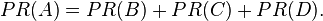$PR(A)= PR(B) + PR(C) + PR(D).\,$

This is 0.75.

Suppose that page B has a link to page C as well as to page A, while page D has links to all three pages. The value of the link-votes is divided among all the outbound links on a page. Thus, page B gives a vote worth 0.125 to page A and a vote worth 0.125 to page C. Only one third of D’s PageRank is counted for A’s PageRank (approximately 0.083).$PR(A)= \frac{PR(B)}{2}+ \frac{PR(C)}{1}+ \frac{PR(D)}{3}.\,$

In other words, the PageRank conferred by an outbound link is equal to the document’s own PageRank score divided by the normalized number of outbound links L( ) (it is assumed that links to specific URLs only count once per document).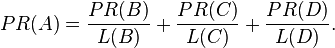$PR(A)= \frac{PR(B)}{L(B)}+ \frac{PR(C)}{L(C)}+ \frac{PR(D)}{L(D)}. \,$

In the general case, the PageRank value for any page u can be expressed as: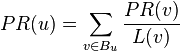$PR(u) = \sum_{v \in B_u} \frac{PR(v)}{L(v)}$,

i.e. the PageRank value for a page u is dependent on the PageRank values for each page v out of the set Bu (this set contains all pages linking to page u), divided by the number L(v) of links from page v.

### Damping factor

The PageRank theory holds that even an imaginary surfer who is randomly clicking on links will eventually stop clicking. The probability, at any step, that the person will continue is a damping factor d. Various studies have tested different damping factors, but it is generally assumed that the damping factor will be set around 0.85.

The damping factor is subtracted from 1 (and in some variations of the algorithm, the result is divided by the number of documents (N) in the collection) and this term is then added to the product of the damping factor and the sum of the incoming PageRank scores. That is,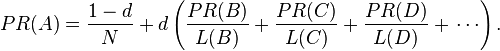$PR(A) = {1 - d \over N} + d \left( \frac{PR(B)}{L(B)}+ \frac{PR(C)}{L(C)}+ \frac{PR(D)}{L(D)}+\,\cdots \right).$

So any page’s PageRank is derived in large part from the PageRanks of other pages. The damping factor adjusts the derived value downward. The original paper, however, gave the following formula, which has led to some confusion:$PR(A)= 1 - d + d \left( \frac{PR(B)}{L(B)}+ \frac{PR(C)}{L(C)}+ \frac{PR(D)}{L(D)}+\,\cdots \right).$

The difference between them is that the PageRank values in the first formula sum to one, while in the second formula each PageRank gets multiplied by N and the sum becomes N. A statement in Page and Brin’s paper that “the sum of all PageRanks is one” and claims by other Google employees support the first variant of the formula above.

Google recalculates PageRank scores each time it crawls the Web and rebuilds its index. As Google increases the number of documents in its collection, the initial approximation of PageRank decreases for all documents.

The formula uses a model of a random surfer who gets bored after several clicks and switches to a random page. The PageRank value of a page reflects the chance that the random surfer will land on that page by clicking on a link. It can be understood as a Markov chain in which the states are pages, and the transitions are all equally probable and are the links between pages.

If a page has no links to other pages, it becomes a sink and therefore terminates the random surfing process. If the random surfer arrives at a sink page, it picks another URL at random and continues surfing again.

When calculating PageRank, pages with no outbound links are assumed to link out to all other pages in the collection. Their PageRank scores are therefore divided evenly among all other pages. In other words, to be fair with pages that are not sinks, these random transitions are added to all nodes in the Web, with a residual probability of usually d = 0.85, estimated from the frequency that an average surfer uses his or her browser’s bookmark feature.

So, the equation is as follows: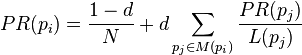$PR(p_i) = \frac{1-d}{N} + d \sum_{p_j \in M(p_i)} \frac{PR (p_j)}{L(p_j)}$

where p1,p2,…,pN are the pages under consideration, M(pi) is the set of pages that link to pi, L(pj) is the number of outbound links on page pj, and N is the total number of pages.

The PageRank values are the entries of the dominant eigenvector of the modified adjacency matrix. This makes PageRank a particularly elegant metric: the eigenvector is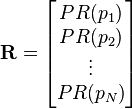$\mathbf{R} = \begin{bmatrix} PR(p_1) \\ PR(p_2) \\ \vdots \\ PR(p_N) \end{bmatrix}$

where R is the solution of the equation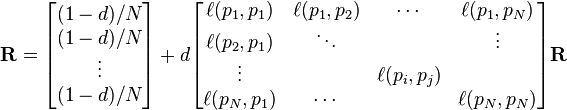$\mathbf{R} = \begin{bmatrix} {(1-d)/ N} \\ {(1-d) / N} \\ \vdots \\ {(1-d) / N} \end{bmatrix} + d \begin{bmatrix} \ell(p_1,p_1) & \ell(p_1,p_2) & \cdots & \ell(p_1,p_N) \\ \ell(p_2,p_1) & \ddots & & \vdots \\ \vdots & & \ell(p_i,p_j) & \\ \ell(p_N,p_1) & \cdots & & \ell(p_N,p_N) \end{bmatrix} \mathbf{R}$

where the adjacency function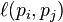$\ell(p_i,p_j)$
is 0 if page pj does not link to pi, and normalized such that, for each i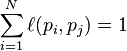$\sum_{i = 1}^N \ell(p_i,p_j) = 1$,

i.e. the elements of each column sum up to 1 (for more details see the computation section below). This is a variant of the eigenvector centrality measure used commonly in network analysis.

Because of the large eigengap of the modified adjacency matrix above, the values of the PageRank eigenvector are fast to approximate (only a few iterations are needed).

As a result of Markov theory, it can be shown that the PageRank of a page is the probability of being at that page after lots of clicks. This happens to equal t − 1 where t is the expectation of the number of clicks (or random jumps) required to get from the page back to itself.

The main disadvantage is that it favors older pages, because a new page, even a very good one, will not have many links unless it is part of an existing site (a site being a densely connected set of pages, such as Wikipedia). The Google Directory (itself a derivative of the Open Directory Project) allows users to see results sorted by PageRank within categories. The Google Directory is the only service offered by Google where PageRank directly determines display order.[citation needed] In Google’s other search services (such as its primary Web search) PageRank is used to weight the relevance scores of pages shown in search results.

Several strategies have been proposed to accelerate the computation of PageRank.

Various strategies to manipulate PageRank have been employed in concerted efforts to improve search results rankings and monetize advertising links. These strategies have severely impacted the reliability of the PageRank concept, which seeks to determine which documents are actually highly valued by the Web community.

Google is known to penalize link farms and other schemes designed to artificially inflate PageRank. In December 2007 Google started actively penalizing sites selling paid text links. How Google identifies link farms and other PageRank manipulation tools are among Google’s trade secrets.

### Computation

To summarize, PageRank can be either computed iteratively or algebraically. The iterative method can be viewed differently as the power iteration method, or power method. It is important to note that the mathematical operations performed in the iterative method and the power method are, in essence, identical.

#### Iterative

In the former case, at t = 0, an initial probability distribution is assumed, usually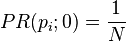$PR(p_i; 0) = \frac{1}{N}$.

At each time step, the computation, as detailed above, yields$PR(p_i;t+1) = \frac{1-d}{N} + d \sum_{p_j \in M(p_i)} \frac{PR (p_j; t)}{L(p_j)}$,

or in matrix notation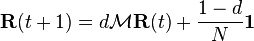$\mathbf{R}(t+1) = d \mathcal{M}\mathbf{R}(t) + \frac{1-d}{N} \mathbf{1}$,       (*)

where$\mathbf{R}_i(t)=PR(p_i; t)$ and$\mathbf{1}$
is the column vector of length N containing only ones.

The matrix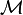$\mathcal{M}$
is defined as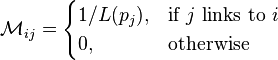$\mathcal{M}_{ij} = \begin{cases} 1 /L(p_j) , & \mbox{if }j\mbox{ links to }i\ \\ 0, & \mbox{otherwise} \end{cases}$

i.e.,$\mathcal{M} := (K^{-1} A)^t$,

where A denotes the adjacency matrix of the graph and K is the diagonal matrix with the outdegrees in the diagonal.

The computation ends when for some small ε$|\mathbf{R}(t+1) - \mathbf{R}(t)| < \epsilon$,

i.e., when convergence is assumed.

#### Algebraic

In the latter case, for$t \to \infty$
(i.e., in the steady state), the above equation (*) reads$\mathbf{R} = d \mathcal{M}\mathbf{R} + \frac{1-d}{N} \mathbf{1}$.       (**)

The solution is given by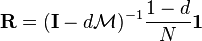$\mathbf{R} = (\mathbf{I}-d \mathcal{M})^{-1} \frac{1-d}{N} \mathbf{1}$,

with the identity matrix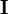$\mathbf{I}$.

The solution exists and is unique for 0 < d < 1. This can be seen by noting that$\mathcal{M}$
is by construction a stochastic matrix and hence has an eigenvalue equal to one because of the Perron-Frobenius theorem.

#### Power Method

If the matrix$\mathcal{M}$
is a transition probability, i.e., column-stochastic with no columns consisting of just zeros and$\mathbf{R}$ is a probability distribution (i.e.,$|\mathbf{R}|=1$,$\mathbf{E}\mathbf{R}=1$
where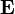$\mathbf{E}$
is matrix of all ones), Eq. (**) is equivalent to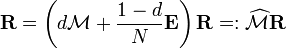$\mathbf{R} = \left( d \mathcal{M} + \frac{1-d}{N} \mathbf{E} \right)\mathbf{R} =: \widehat \mathcal{M} \mathbf{R}$.       (***)

Hence PageRank$\mathbf{R}$
is the principal eigenvector of$\widehat\mathcal{M}$.
A fast and easy way to compute this is using the power method: starting with an arbitrary vector x(0), the operator$\widehat\mathcal{M}$
is applied in succession, i.e.,$x(t+1) = \widehat\mathcal{M} x(t)$,

until

| x(t + 1) − x(t) | < ε.

Note that in Eq. (***) the matrix on the right-hand side in the parenthesis can be interpreted as$\frac{1-d}{N} \mathbf{I} = (1-d)\mathbf{P} \mathbf{1}^t$,

where$\mathbf{P}$
is an initial probability distribution. In the current case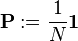$\mathbf{P} := \frac{1}{N} \mathbf{1}$.

Finally, if$\mathcal{M}$
has columns with only zero values, they should be replaced with the initial probability vector$\mathbf{P}$.
In other words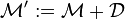$\mathcal{M}^\prime := \mathcal{M} + \mathcal{D}$,

where the matrix$\mathcal{D}$
is defined as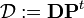$\mathcal{D} := \mathbf{D} \mathbf{P}^t$,

with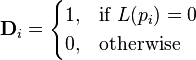$\mathbf{D}_i = \begin{cases} 1, & \mbox{if }L(p_i)=0\ \\ 0, & \mbox{otherwise} \end{cases}$

In this case, the above two computations using$\mathcal{M}$
only give the same PageRank if their results are normalized: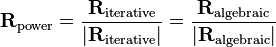$\mathbf{R}_{\textrm{power}} = \frac{\mathbf{R}_{\textrm{iterative}}}{|\mathbf{R}_{\textrm{iterative}}|} = \frac{\mathbf{R}_{\textrm{algebraic}}}{|\mathbf{R}_{\textrm{algebraic}}|}$.

#### Efficiency

Depending on the framework used to perform the computation, the exact implementation of the methods, and the required accuracy of the result, the computation time of the these methods can vary greatly. Usually if the computation has to be performed many times (i.e., for growing networks) or the network size is large, the algebraic computation is slower and more memory hungry due to the inversion of the matrix.

### Variations

The Google Toolbar‘s PageRank feature displays a visited page’s PageRank as a whole number between 0 and 10. The most popular websites have a PageRank of 10. The least have a PageRank of 0. Google has not disclosed the precise method for determining a Toolbar PageRank value. The displayed value is not the actual value Google use so it is only a rough guide. ‘Toolbar’ PageRank is different than Google PageRank because the PageRank displayed in the toolbar is not 100% reflective of the way Google judges the value of a website.

The Google Toolbar’s PageRank is updated approximately 4 times a year, so often shows out of date values. It was last updated on 3 April 2010.

### SERP Rank

The SERP (Search Engine Results Page) is the actual result returned by a search engine in response to a keyword query. The SERP consists of a list of links to web pages with associated text snippets. The SERP rank of a web page refers to the placement of the corresponding link on the SERP, where higher placement means higher SERP rank. The SERP rank of a web page is not only a function of its PageRank, but depends on a relatively large and continuously adjusted set of factors (over 200), commonly referred to by internet marketers as “Google Love”. SEO (Search Engine Optimization) is aimed at achieving the highest possible SERP rank for a website or a set of web pages.

With the introduction of Google Places into the mainstream organic SERP, PageRank plays little to no role in ranking a business in the Local Business Results. Albeit the theory of citations is still computed in their algorithm, PageRank is not a factor since Google ranks business listings and not web pages.

### Google directory PageRank

The Google Directory PageRank is an 8-unit measurement. These values can be viewed in the Google Directory. Unlike the Google Toolbar which shows the PageRank value by a mouseover of the green bar, the Google Directory does not show the PageRank as a numeric value but only as a green bar.

### Other uses

A version of PageRank has recently been proposed as a replacement for the traditional Institute for Scientific Information (ISI) impact factor, and implemented at eigenfactor.org. Instead of merely counting total citation to a journal, the “importance” of each citation is determined in a PageRank fashion.

A similar new use of PageRank is to rank academic doctoral programs based on their records of placing their graduates in faculty positions. In PageRank terms, academic departments link to each other by hiring their faculty from each other (and from themselves).

PageRank has been used to rank spaces or streets to predict how many people (pedestrians or vehicles) come to the individual spaces or streets. In lexical semantics it has been used to perform Word Sense Disambiguation and to automatically rank WordNet synsets according to how strongly they possess a given semantic property, such as positivity or negativity.

A dynamic weighting method similar to PageRank has been used to generate customized reading lists based on the link structure of Wikipedia.

A Web crawler may use PageRank as one of a number of importance metrics it uses to determine which URL to visit during a crawl of the web. One of the early working papers  which were used in the creation of Google is Efficient crawling through URL ordering , which discusses the use of a number of different importance metrics to determine how deeply, and how much of a site Google will crawl. PageRank is presented as one of a number of these importance metrics, though there are others listed such as the number of inbound and outbound links for a URL, and the distance from the root directory on a site to the URL.

The PageRank may also be used as a methodology to measure the apparent impact of a community like the Blogosphere on the overall Web itself. This approach uses therefore the PageRank to measure the distribution of attention in reflection of the Scale-free network paradigm.

In any ecosystem, a modified version of PageRank may be used to determine species that are essential to the continuing health of the environment.

### Google’s rel="nofollow" option

In early 2005, Google implemented a new value, “nofollow“, for the rel attribute of HTML link and anchor elements, so that website developers and bloggers can make links that Google will not consider for the purposes of PageRank — they are links that no longer constitute a “vote” in the PageRank system. The nofollow relationship was added in an attempt to help combat spamdexing.

As an example, people could previously create many message-board posts with links to their website to artificially inflate their PageRank. With the nofollow value, message-board administrators can modify their code to automatically insert “rel=’nofollow'” to all hyperlinks in posts, thus preventing PageRank from being affected by those particular posts. This method of avoidance, however, also has various drawbacks, such as reducing the link value of legitimate comments. (See: Spam in blogs#nofollow)

In an effort to manually control the flow of PageRank among pages within a website, many webmasters practice what is known as PageRank Sculpting – which is the act of strategically placing the nofollow attribute on certain internal links of a website in order to funnel PageRank towards those pages the webmaster deemed most important. This tactic has been used since the inception of the nofollow attribute, but the technique has been thought by many to have lost its effectiveness.

### Removal from Google Webmaster Tools

On October 15, 2009, Google employee Susan Moskwa confirmed that the company had removed PageRank from its Webmaster Tools section. Her post said in part, “We’ve been telling people for a long time that they shouldn’t focus on PageRank so much; many site owners seem to think it’s the most important metric for them to track, which is simply not true.”